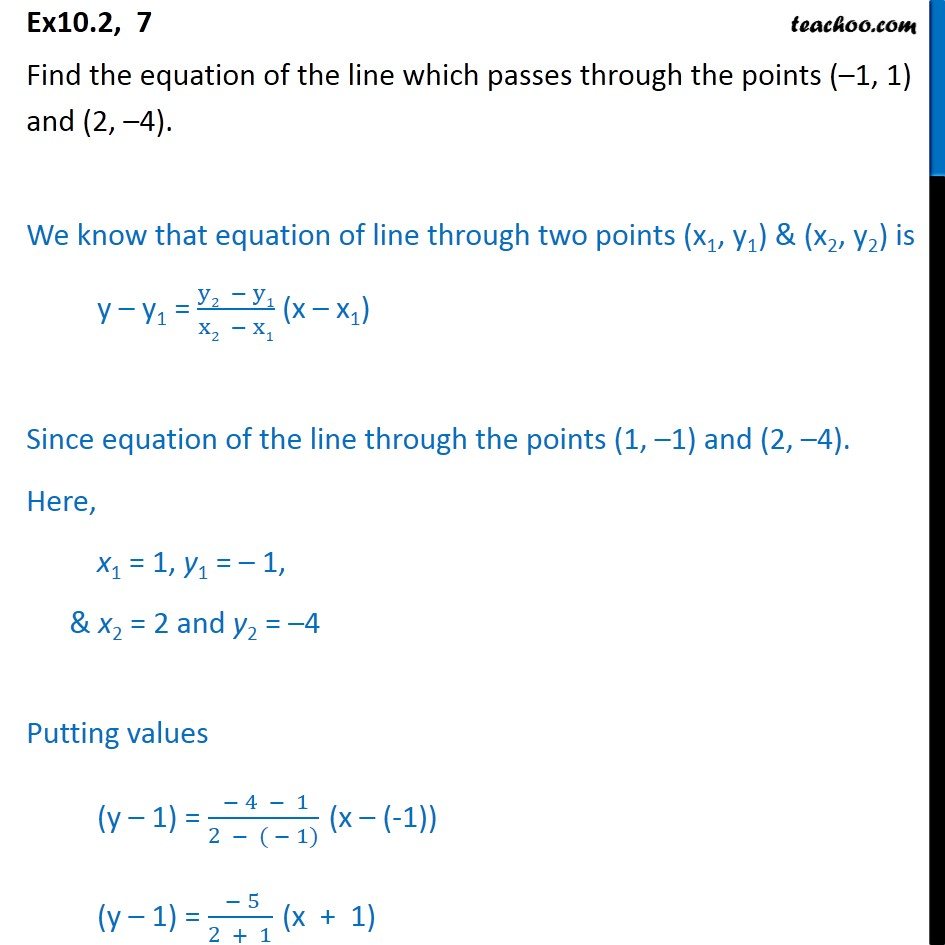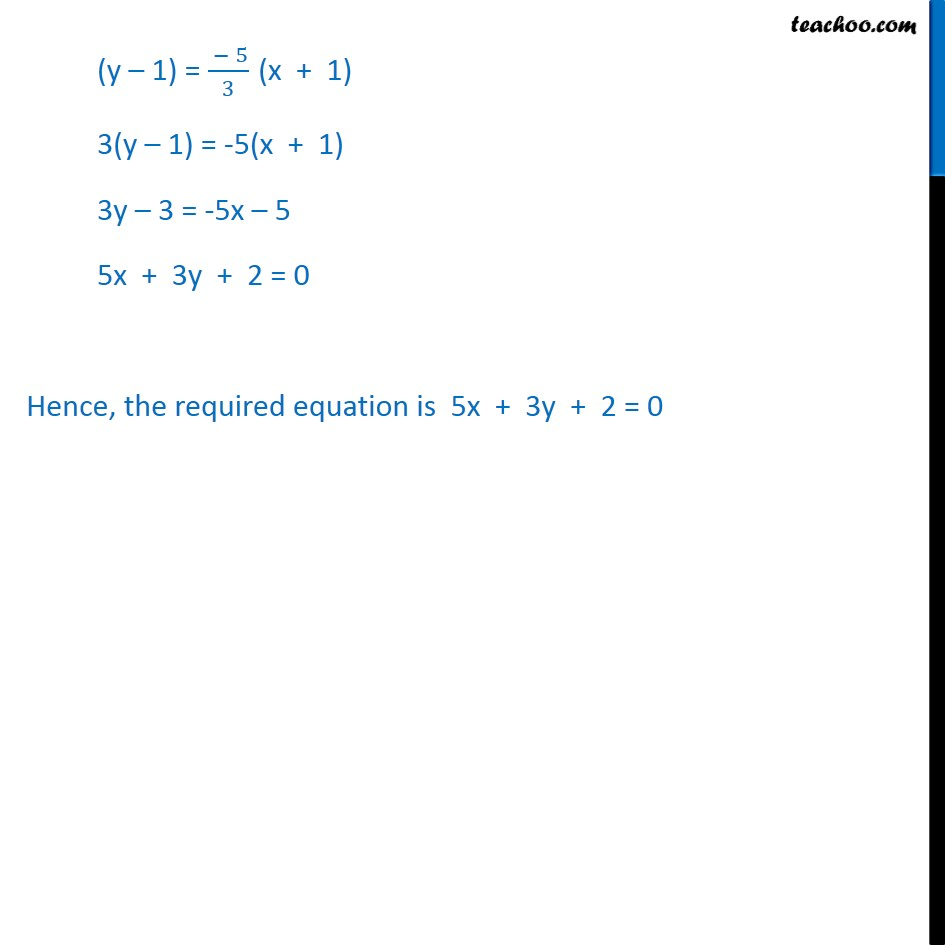1. Chapter 10 Class 11 Straight Lines
2. Serial order wise
3. Ex 10.2

Transcript

Ex10.2, 7 Find the equation of the line which passes through the points ( 1, 1) and (2, 4). We know that equation of line through two points (x1, y1) & (x2, y2) is y y1 = (y2 y1)/(x2 x1) (x x1) Since equation of the line through the points (1, 1) and (2, 4). Here, x1 = 1, y1 = 1, & x2 = 2 and y2 = 4 Putting values (y 1) = ( 4 1)/(2 ( 1)) (x (-1)) (y 1) = ( 5)/(2 + 1) (x + 1) (y 1) = ( 5)/3 (x + 1) 3(y 1) = -5(x + 1) 3y 3 = -5x 5 5x + 3y + 2 = 0 Hence, the required equation is 5x + 3y + 2 = 0

Ex 10.2